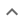•# Linear equations with one unknown

We think you wrote:

###This solution deals with linear equations with one unknown.

Solution foundf=-1/3=-0.333

## Step by Step Solution### Rearrange:

Rearrange the equation by subtracting what is to the right of the equal sign from both sides of the equation :

(7-3*f)/4-(2)=0

## Step  1  :

            7 - 3f
Simplify   ——————
4


#### Equation at the end of step  1  :

  (7 - 3f)
———————— -  2  = 0
4


## Step  2  :

#### Rewriting the whole as an Equivalent Fraction :

Subtracting a whole from a fraction

Rewrite the whole as a fraction using  4  as the denominator :

         2     2 • 4
2 =  —  =  —————
1       4


Equivalent fraction : The fraction thus generated looks different but has the same value as the whole

Common denominator : The equivalent fraction and the other fraction involved in the calculation share the same denominator

#### Adding fractions that have a common denominator :

Adding up the two equivalent fractions
Add the two equivalent fractions which now have a common denominator

Combine the numerators together, put the sum or difference over the common denominator then reduce to lowest terms if possible:

 (7-3f) - (2 • 4)     -3f - 1
————————————————  =  ———————
4                4


## Step  3  :

#### Pulling out like terms :

Pull out like factors :

-3f - 1  =   -1 • (3f + 1)

#### Equation at the end of step  3  :

  -3f - 1
———————  = 0
4


## Step  4  :

#### When a fraction equals zero :

 4.1    When a fraction equals zero ...

Where a fraction equals zero, its numerator, the part which is above the fraction line, must equal zero.

Now,to get rid of the denominator, Tiger multiplys both sides of the equation by the denominator.

Here's how:

  -3f-1
————— • 4 = 0 • 4
4


Now, on the left hand side, the  4  cancels out the denominator, while, on the right hand side, zero times anything is still zero.

The equation now takes the shape :
-3f-1  = 0

#### Solving a Single Variable Equation :

Solve  :    -3f-1 = 0

Add  1  to both sides of the equation :

-3f = 1
Multiply both sides of the equation by (-1) :  3f = -1

Divide both sides of the equation by 3:
f = -1/3 = -0.333

## One solution was found :

f = -1/3 = -0.333